Monday, March 27, 2023

## Grade 12 Vertical Projectile Motion in One Dimension

#### 1. Introduction

In this chapter we are going to look at the motion of objects that are either projected, thrown, or shot directly into the air, be it vertically upwards, downwards or when objects are dropped. This is something that everyone has experienced while playing games and we all have a strong intuition that ‘what goes up, must come down’, but now we’ll study why that is the case.

• Units and unit conversions — Physical Sciences, Grade 10, Science skills

• Rectilinear motion — Physical Sciences, Grade 10, Motion in one dimension

• Linear equations — Mathematics, Grade 10, Equations and inequalities

#### 2. Vertical Projectile Motion

In Grade 11, we studied the motion of objects in free fall and saw that such an object has a constant gravitational acceleration of​​ g. We now study the motion of objects that are moving upwards or downwards while experiencing a force due to gravity. We call this projectile motion. We will only consider the case where objects move vertically upwards and/or downwards – meaning that there is no horizontal displacement of the object, only vertical displacement.​​ When we talk about a projectile we mean a body or particle that is project or launched​​ and then moves under the inﬂuence of only gravity.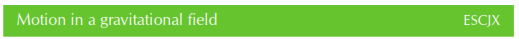We know from Newton’s Law of Universal Gravitation that an object in the Earth’s gravitational ﬁeld experiences a force pulling it towards the centre of the Earth. If this is the only force acting on the object then the object will accelerate towards the centre of the Earth. A person standing on the Earth’s surface will interpret this acceleration as objects always falling downwards. In Grade 11, we showed that the value of the acceleration due to gravity can be treated as a having a constant magnitude of g = 9,8 m.s-2

IMPORTANT!

In reality, if you go very far away from the Earth’s surface, the magnitude of​​ g​​ would change, but, for everyday problems, we can safely treat it as constant. We also ignore any effects that air resistance (drag) might have.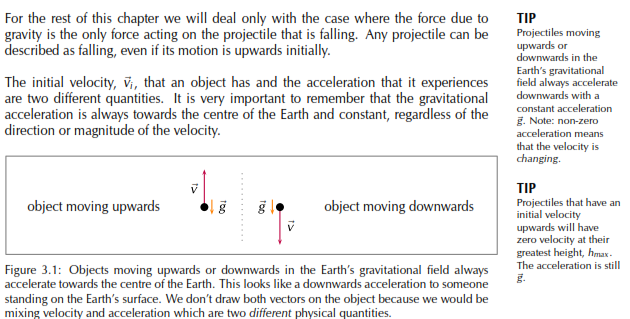This means that if an object is moving upwards with some initial velocity in the vertical direction, the magnitude of the velocity in the vertical direction decreases until it stops (v = 0 m.s-1​​ ) for an instant. The point at which the velocity is reduced to zero corresponds with the maximum height,​​ hmax​​ , that the object reaches. After this, the object starts to fall. It is very important to remember that the acceleration is constant but the​​ velocity vector has changed in magnitude and direction. At the maximum height where the velocity is zero the acceleration is still​​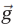​​ .​​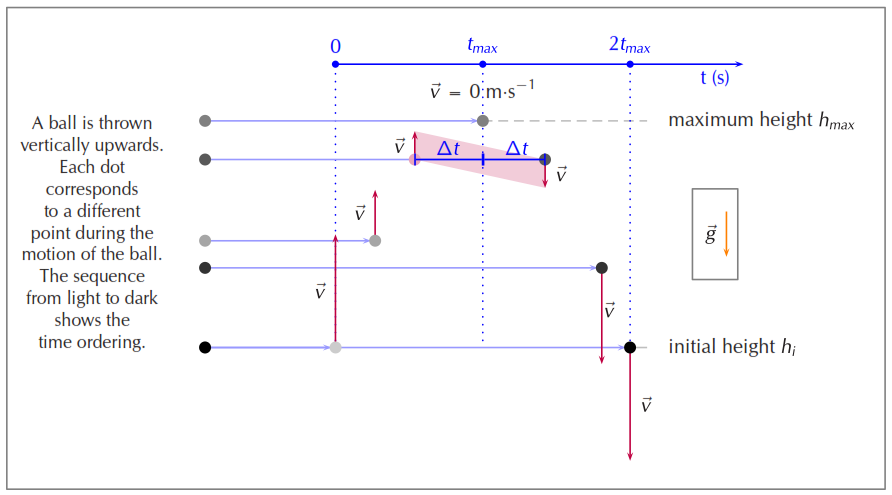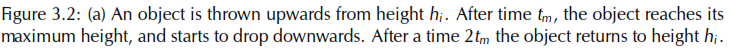Consider an object thrown upwards from a vertical height h.​​ We have said that the​​ object will travel upwards with decreasing vertical velocity until it stops, and then starts​​ moving vertically downwards. The time that it takes for the object to fall back down​​ to height​​ hi​​ is the same as the time taken for the object to reach its maximum height​​ from height hi. This is known as time symmetry. This is a​​ consequence​​ of the uniform​​ acceleration the projectile experiences.​​ This has two implications for projectiles that pass through a point on both the upward​​ and downward part of their motion when in free-fall:

• time symmetry: the time intervals during the upward motion and the downward motion are the same, for example it will take the same time to rise from initial position to maximum height as it will to drop back to the initial position. This applies to any point as shown by the pink dot in Figure 3.2. The time interval​​ between the projectile passing the point and being at maximum height is the same,​​ t.

• magnitude of velocity: the magnitude of the velocity at the same point on the​​ upward and downward motion will be the same, the direction will be reversed,​​ as indicated by the shaded region in Figure 3.2.

This is very useful when solving problems because if you have any information about the upward motion you can learn something about the downward motion as well and vice versa.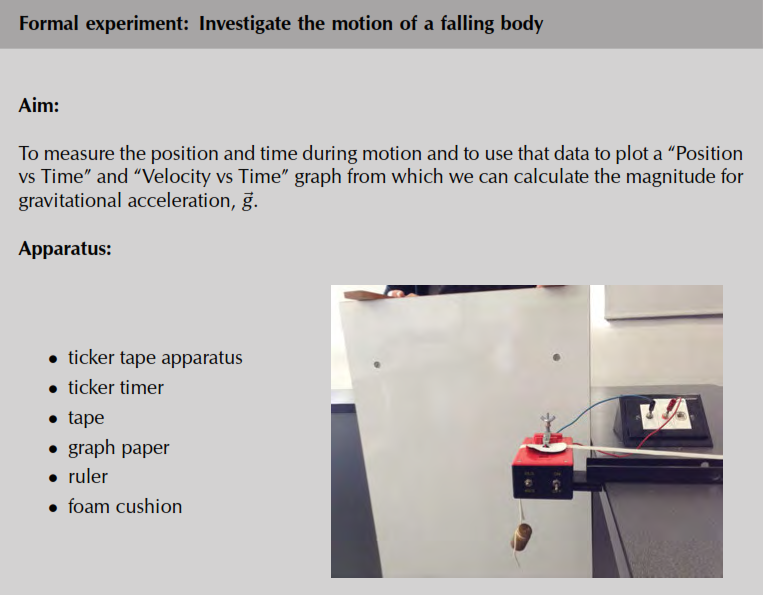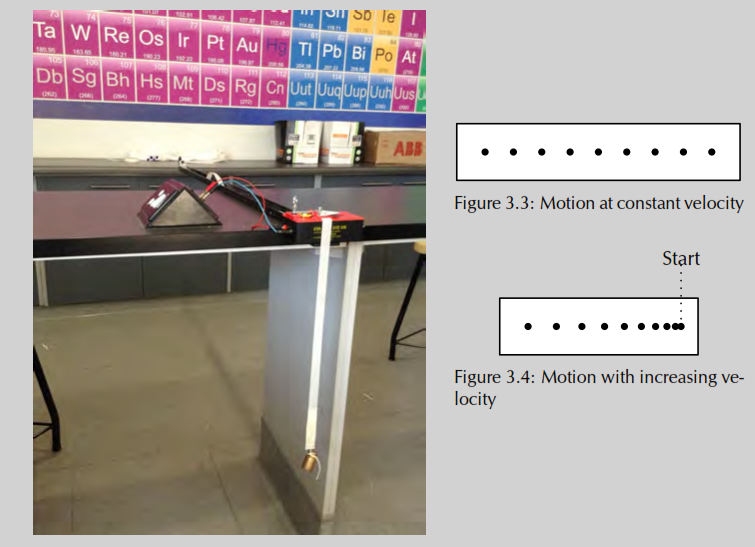Method:

1. Work with a friend. Copy the table below into your workbook.

2. Clamp the timer to the table to allow the tape to fall through the staples on the​​ timer such that the ticker tape will be perpendicular to the ﬂoor. Plug in the​​ timer.

3. Thread a piece of ticker tape through the staples. Make sure the tape is under the carbon disc. The length of the tape should be the distance from the timer to the ﬂoor.

4. Using masking tape attach a 100 g mass to the end of the tape closest to the ﬂoor.

5. Position the foam cushion directly below the mass. (The cushion will protect your masses and your ﬂoor)

6. Hold the tape so that it is vertical and will easily pass through the staples.

7. Turn on the timer.

8. Let go of the tape with the mass, allowing it to drop to the ﬂoor.

9. Turn off the timer.

10. Repeat.

11. Take each tape and ﬁnd the ﬁrst clear dot nearest the mass end of the tape. Circle​​ this ﬁrst clear dot and label it as zero. Then count ﬁve dots and label the ﬁfth dot​​ as number 1. Count another 5 dots and label the ﬁfth dot number 2. Continue​​ this procedure.

12. On each piece of tape, measure the distance between successive dots. Note these distances in the table below.

13. Use the frequency of the ticker timer to work out the time intervals between successive dots. Note these times in the table below.

14. Work out the average of the two distance values between successive numbered dots.

15. The time interval between two dots is 0,02 s so the time between numbered dots is 0,1 s.

16. Use the average distance between numbered dots and the known time interval to calculate velocity.

17. Use the magnitude of the velocity and time values to plot a graph of “Velocity vs Time” onto graph paper. Stick the graph paper into your workbook.

18. Draw the best straight line through your data points.

19. Determine the slope of the straight line. This is the magnitude of the acceleration.